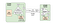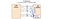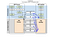# A Framework for Efficient STARKs

## Combining probabilistic proofs and hash functions

This is the fifth and final post in our STARK Math series.

In our first post we introduced the notion of inclusive accountability, and its importance for permissionless blockchains. We also explained why the scalability properties of STARKs enable achieving this notion. We recommend the reader to read this first post before proceeding.

In our second, third, and fourth posts we dove into the mathematical theory behind STARKs. We explained how to transform computational statements into polynomial constraints. We explained how to reduce checking polynomial constraints to checking low-degreeness. And we described an efficient test for low-degreeness. Below we do not assume familiarity with these posts, except for a single section that explains how protocols described in these posts can be viewed as a certain type of probabilistic proof (whose details do not matter in this post).

In this post we conclude the series, by revisiting the notion of a STARK and then explaining how to construct a STARK from probabilistic proofs and cryptographic hash functions.

## Our goal: scalable transparent arguments

We wish to construct a STARK, which is a cryptographic proof (aka an “argument”) that is both scalable and transparent. Our first post describes this goal in detail, which we now summarize.

We consider cryptographic proofs for Computational Integrity (CI) statements. This means that, for a given program 𝐀, input x, output y, and time bound T, we wish to produce a proof string 𝛑 attesting to the statement

“𝐀(x) outputs y within T computation steps”.

No (efficient) procedure should be able to produce valid-looking proofs for false statements (e.g., claiming 𝐀(x)=1 when instead 𝐀(x)=0). More general CI statements are possible, where 𝐀 additionally takes a private auxiliary input, but we ignore this in this post.

STARKs are cryptographic proofs that satisfy the following desirable properties.

• Scalability: the time needed to produce 𝛑 is T·polylog(T), whereas the time needed to validate 𝛑 is only polylog(T); in particular, the length of 𝛑 is polylog(T). In other words, producing proofs is not much more expensive than just running the original computation, and validating proofs is exponentially faster than running the original computation. Proofs are exponentially shorter than the size of the original computation.
• Transparency: the global parameters of the cryptographic proof system, used to produce and validate proofs, have no trapdoors. This is in contrast to proof systems that rely on a trusted party to sample public parameters based on secret information, which can be used as a “trapdoor” to compromise the security of the proof system.

By the end of this post, you will have an informal understanding of where the properties of scalability and transparency come from.

We briefly note here that STARKs only make use of lightweight symmetric cryptography (cryptographic hash functions), which makes them fast and (plausibly) post-quantum secure. This is in contrast to many other cryptographic proofs, whose security relies on public-key cryptography that is both expensive and insecure against quantum adversaries.

Known STARK constructions combine two ingredients:

1. long proofs that can be verified via random, and inexpensive, local checks; and
2. a cryptographic hash function such as SHA-256 or Keccak.

Informally, the first component gives a STARK its scalability, while the second component gives a STARK its transparency (without impeding scalability). In the next few sections we elaborate on the above blueprint.

• First, we describe the Micali construction, a method to construct cryptographic proofs that follows the above blueprint. The proof systems that underlie this construction are known as probabilistically-checkable proofs (PCPs). We describe this construction for pedagogical reasons: it is an elegant special case of the construction that we use.
• Second, we explain why the Micali construction provides transparency and scalability.
• Third, we describe the notion of Interactive Oracle Proofs (IOPs), which generalizes PCPs and enables designing much more efficient protocols. We also explain how the arithmetization and low-degree testing protocols in prior posts are IOPs.
• Finally, we mention the BCS construction, an extension of the Micali construction that uses the more general notion of IOPs instead of PCPs. Efficient STARKs, including those that we use, are obtained via the BCS construction.

## Cryptographic proofs from PCPs, via the Micali construction

In this section we introduce probabilistically-checkable proofs (PCPs), a type of proof system that involves a random local check to a long proof. Then we explain how a PCP can be combined with lightweight cryptography to obtain a STARK. This construction comes from a paper of Silvio Micali (building on prior work of Joe Kilian).

A probabilistically-checkable proof (PCP) is a protocol between a PCP prover and a PCP verifier that enables establishing the correctness of Computational Integrity (CI) statements via a random local check to a long proof. Given a CI statement (𝐀,x,y,T), the PCP prover produces a proof string 𝚿 that “encodes” the computation trace of the CI statement. While the proof 𝚿 is longer than the T-step computation trace (𝚿’s length is quasilinear in T), 𝚿 has the remarkable feature that it can be validated via a probabilistic test that reads only a small part of 𝚿. Namely, given the same CI statement (A,x,y,T), the PCP verifier can validate 𝚿 by randomly reading a small number of locations of 𝚿 and then running an inexpensive “local check” on the read values. (The number of read locations can be a small constant, like 3, independent of T!) If the CI statement is true then the verifier always accepts. If instead the CI statement is false, then the verifier rejects with high probability, regardless of how the proof string 𝚿 was chosen. See Figure 2 for a diagram.

Recall that our goal is to produce proofs 𝛑 that are short and fast to validate. This is quite different from PCPs, which instead involve inexpensive local checks to long proofs 𝚿. So how do we go from 𝚿 to 𝛑?

A natural idea that may come to mind is to have the prover pre-sample a local view of 𝚿, on behalf of the verifier, and then send this local view as the proof 𝛑. In more detail, the prover simulates a random local check of the PCP verifier on the long proof 𝚿 and then includes in a proof 𝛑 only locations of 𝚿 read via this local check; the prover also includes in 𝛑 the randomness 𝛒 used for the PCP verifier. (Note that 𝛑 is short because the number of read locations of 𝚿 is small.) The intuition of pre-sampling is that, in order to validate 𝛑, one could run the PCP verifier with the very same randomness 𝛒, which would cause reading the very same locations of 𝚿, which were included in 𝛑. This appealing idea, however, is flawed. First, a cheating prover may include in 𝛑 a choice of “randomness” 𝛒 that, in fact, is not random. Second, a cheating prover may choose answers to the PCP verifier queries that depend on 𝛒 in order to pass the PCP verifier’s local check. This is possible because the security of a PCP relies on 𝚿 being immutable, i.e., fixed before the random local check of the verifier is chosen.

The above problems can be addressed by using any cryptographic hash function H such as SHA-256 (modeled as a random oracle), to realize a secure pre-sampling. Here “secure” means that the prover will be able to convince the verifier that the information included in the short proof 𝛑 is an “honest” random local check that was ran on some long proof 𝚿.

Informally, as depicted in Figure 3, the prover is expected to use the hash function H to commit to the long proof 𝚿 via a Merkle tree, and then derive the randomness 𝛒 by using H to hash the root of the Merkle tree. This ensures that the randomness 𝛒 is “random-like” (since 𝛒 is an output of the hash function H) and also ensures that 𝛒 is chosen after the prover has committed to 𝚿. Now the prover can do pre-sampling as described above, namely, it simulates a random local check of the PCP verifier on randomness 𝛒, to determine which locations of 𝚿 should be included in 𝛑. Finally, the prover “decommits” to the chosen locations, by including in 𝛑 an authentication path for each chosen location (the authentication path for a location consists of the siblings of nodes on the path from the location to the root). The authentication paths demonstrate that the claimed values of 𝚿 are consistent with the Merkle root, and in particular were not chosen by the prover after the randomness 𝛒 was derived from the root. Overall, the short proof 𝛑 will only include the claimed root of the Merkle tree, the claimed values of 𝚿 for the selected locations, and authentication paths for each of these values (relative to the root). One can then validate 𝛑 by checking all the authentication paths for the claimed values against the root, re-deriving the randomness 𝛒 from the root, and checking that the PCP verifier accepts the claimed values when run with the randomness 𝛒.¹

To summarize, we have used the hash function H to realize a “secure pre-sampling” that enables including in the short proof 𝛑 a single random local check of the long proof 𝚿.

: This “justification” for why secure pre-sampling works is just intuition, and obtaining a formal proof of security requires some work. For example, a malicious prover could try to commit to many different proofs 𝚿 in search for a “favorable” choice of randomness 𝛒, and then include this favorable choice in 𝛑. A proof of security would have to establish that such provers, and indeed any efficient prover, will fail with high probability.Figure 3: Diagram of the Micali construction, which uses a cryptographic hash function to securely pre-sample a PCP.

## Transparency comes from the cryptography

An important feature of the Micali construction is that the only cryptography needed for producing or validating a short proof 𝛑 is a cryptographic hash function H (e.g., SHA-256 or Keccak). The choice of H is thus the only “global parameter” that all users of the proof system need to know, and this choice can be made via public information. This means that cryptographic proofs obtained via the Micali construction are transparent.

The foregoing is in contrast to other cryptographic proofs wherein producing or validating proofs requires using global parameters that are produced based on secret information. For those familiar with cryptographic proofs based on pairings, a typical example of global parameters is

(G, 𝛂·G, 𝛂²·G, 𝛂³·G, …)

where G is a group element and 𝛂 is a secret scalar. Such global parameters must be sampled by a trusted party, or via a multi-party ceremony because users should not know the “trapdoor” 𝛂. Indeed, knowing 𝛂 would enable producing valid-looking proofs for false statements.

## Scalability comes from the probabilistic proof

Another important feature of the Micali construction is that the time to produce/validate the short proof 𝛑 is close to the time to produce/validate the long proof 𝚿. This is simply because the needed cryptographic operations are inexpensive when compared to the PCP operations. We thus learn that efficiency in the Micali construction is essentially determined by the efficiency of the underlying PCP. In particular, if the PCP is scalable (producing 𝚿 takes time quasilinear in T whereas validating 𝚿 is exponentially faster) then the Micali construction yields a scalable cryptographic proof. Constructions of scalable PCPs are known (see this paper).

Unfortunately, the costs of PCPs remain very high, which makes them unfit for practical use. Because of this, implementations of STARKs are not based on PCPs via the Micali construction. Instead, they are based on another type of probabilistic proof, for which one can achieve scalability, with good concrete costs and even with zero knowledge. We discuss this next.

## IOPs: a new notion of probabilistic proof

Efficient STARKs are based on a type of probabilistic proof system known as interactive oracle proofs (IOPs), which was introduced in 2015. Informally, a prover and a verifier engage in an interactive protocol where in each round the verifier sends some randomness 𝛔ᵢ to the prover, and the prover replies with a long proof 𝚿ᵢ. At the end of the interaction, the verifier performs a random local check on all the long proofs (𝚿₁,𝚿₂,…) sent by the prover throughout the interaction. See Figure 4 for a diagram. Note that a PCP is simply a “non-interactive IOP”, and thus is a restricted case.Figure 4: Diagram of an interactive oracle proof (IOP). This notion extends the notion of a PCP (cf. Figure 2).

In the last few years, researchers have developed numerous design principles for constructing highly-efficient IOPs: [BCGV16], [BCGRS16], [BB+16], [BBGR16], [BCFGRS16], [BBHR17], [BBHR18], [BCRSVW18], [BKS19], [BGKS19]. The IOP protocol that we use in our STARK constructions is most closely related to [BBHR18].

## Prior posts describe an efficient IOP

We now explain how our prior posts on arithmetization and low-degree testing actually described an efficient IOP. In Figure 5 we provide a diagram of this IOP. The first phase of the protocol is arithmetization, which transforms the given CI statement (𝐀,x,y,T) into a problem that involves establishing degree bounds on certain polynomials. Second phase is low-degree testing, which solves this latter problem. We summarize the protocol’s workflow.

Arithmetization (blue area in Figure 5). The prover and verifier transform the program 𝐀 into a collection of polynomial constraints, as described in our post Arithmetization I. In addition, the prover runs the computation described by (𝐀,x,y,T), obtaining a T-step computation trace.

Then, as described in our post Arithmetization II, the prover encodes this trace to obtain an encoded trace 𝚽, which is sent to the verifier. (Here the prover does not need to receive any randomness from the verifier before sending 𝚽.) After that, the verifier sends randomness 𝛔₀ which enables both the prover and verifier to “bundle” all the polynomial constraints into a single polynomial constraint, by taking their random linear combination. The prover combines this latter with the encoded trace 𝚽 in order to obtain a composed polynomial 𝚵, which is sent to the verifier. The verifier ensures via a local consistency check that 𝚽 and 𝚵 are suitably related. At this point, if the local consistency check passes with high probability, the CI statement is true if and only if 𝚽 and 𝚵 have low-degree.

Low-degree testing (gray area in Figure 5). The prover uses the FRI protocol (described in our low-degree testing post) to convince the verifier that 𝚽 and 𝚵 are evaluations of low-degree polynomials. This involves engaging in a protocol where in each round the verifier sends some randomness 𝛔ᵢ and the prover replies with an auxiliary proof 𝚿ᵢ, and at the end of the protocol the verifier performs a random local check on 𝚽,𝚵 and the 𝚿ᵢ’s. If the FRI protocol’s verifier accepts with high probability then 𝚽 and 𝚵 have the desired degrees. If so, the verifier concludes that the CI statement (𝐀,x,y,T) is a true statement.Figure 5: Diagram of the IOP protocol described in prior posts.

## Cryptographic proofs via the BCS construction

Efficient STARKs are obtained via the BCS construction a method that combines an IOP with a cryptographic hash function H in order to obtain a cryptographic proof. This method is an extension of the Micali construction, and it retains its features (of transparency and scalability) that we discussed above. We do not describe the BCS construction in this post, and only remark that it can be viewed as applying the Micali construction at each round of an IOP and putting each round’s commitments in a hash chain (which keeps the rounds in order).

# Conclusion

In this post we have explained that efficient STARK constructions are obtained by combining efficient IOPs (a type of probabilistic proof) and cryptographic hash functions. The IOP confers the STARK its scalability, while the hash function confers the STARK its transparency. Different STARK constructions differ in the underlying IOPs, and we have explained how our prior posts have described the components of the IOP protocol used in our STARK construction.

This concludes our STARK Math series. We hope that it was valuable to you!

Alessandro Chiesa and Gideon Kaempfer

## StarkWare

Developing the Full Proof Stack for STARK

## StarkWare

Developing the Full Proof Stack for STARK

Written by

## StarkWare

bringing scalability and privacy to a blockchain near you## StarkWare

Developing the Full Proof Stack for STARK

## More From Medium

Medium is an open platform where 170 million readers come to find insightful and dynamic thinking. Here, expert and undiscovered voices alike dive into the heart of any topic and bring new ideas to the surface. Learn more

Follow the writers, publications, and topics that matter to you, and you’ll see them on your homepage and in your inbox. Explore

If you have a story to tell, knowledge to share, or a perspective to offer — welcome home. It’s easy and free to post your thinking on any topic. Write on Medium